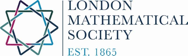# 7th OPSFOTAThis meeting is part of the activities of the Research Group on Orthogonal Polynomials, Special Functions and Operator Theory and Applications (OPSFOTA).

This seventh meeting will take place at KU Leuven in Belgium, on the 6th September 2019. The meeting is supported by a London Mathematical Society Joint Research Groups grant.

Speakers:

Tom Claeys (Louvain-la-Neuve, Belgium),
Daan Huybrechs (Leuven, Belgium),
Marina Iliopoulou (Kent, UK),
Ana Matos (Lille University, France)

Registration: no registration is necessary, the meeting is free for everyone to attend.

Arno Kuijlaars (arno.kuijlaars@kuleuven.be)
or
Walter Van Assche (walter.vanassche@kuleuven.be)

Venue: Building N of campus KU Leuven – Arenberg III, Celestijnenlaan 200, Heverlee

TIMETABLE  – All talks take place in Building N of campus KU Leuven – Arenberg III, Celestijnenlaan 200, Heverlee

Title: Matrix valued orthogonal polynomials related to SU(n+1)
12.00-12.50:  Ana Matos
Title: A rational approach for solving a class of equilibrium problems in potential theory
13.00 -14.30 Lunch
14.30-15.20: Marina Iliopoulou
Title: Sharp estimates for Hö​rmander-type operators
15.30-16.00: Coffee/Tea break
16.00-16.50: Tom Claeys
Title: The lower tail of the KPZ equation via a Riemann-Hilbert approach
17.00-17.50: Daan Huybrechs
Title: Orthogonal polynomials in the complex plane and Gaussian quadrature
18.30: Dinner

Titles and Abstracts
Title: Matrix valued orthogonal polynomials related to SU(n+1)
Abstract: The relation between representation theory and special functions is a fundamental one. Multivariable
functions occuring as characters on $SU(n+1)$, which are Chebyshev polynomials for $n=1$, can be realised as spherical functions. The spherical functions are interpreted on the product group $G=SU(n+1) \times SU(n+1)$ with the diagonal subgroup $K$ as the fixed points of the involution that flips the order in the product. Now the spherical functions can be interpreted as functions on $G$ that are left and right invariant with respect to $K$. Upon replacing the left and right invariance with respect to $K$ by another, nontrivial, representation we obtain matrix valued spherical functions.
From these matrix valued spherical functions we show how to build matrix valued multivariable orthogonal polynomials. The number of variables is $n$ and the size of the matrix is of specific size, but can become arbitrarily large. For the cases $n=2$, $n=3$ we state some explicit cases, and in case of $n=2$ we obtain matrix valued analogues of Koornwinder’s orthogonal polynomials on Steiner’s hypocycloid.
This is joint work with Maarten van Pruijssen en Pablo Román.
Ana Matos
Title: A rational approach for solving a class of equilibrium problems in potential theory
Abstract: We are interested in computing the unknown density of an equilibrium
problem in logarithmic potential theory where the support of the
equilibrium measure is a finite union of distinct intervals. Inspired by
a Riemann-Hilbert approach, we reduce the problem to the solution of
a system of singular integral equations with Cauchy kernels.
After briefly recalling the well studied polynomial approach , we will
be interested in considering rational approximations of the solutions,
expressed in a basis of orthogonal rational functions with prescribed
poles. This new approach ensures stable computations. These approximations
satisfy some interpolatory conditions. Inspired by the third
Zolotareff problem, the poles and the interpolation points are chosen
in such a way that we can ensure small errors. We will also discuss a
link with recent performant algorithms like the multipole method.
Finally our new error estimates will be confirmed by numerical
results.
This is a joint work with Bernd Beckermann.
Marina Iliopoulou
Title: Sharp estimates for Hö​rmander-type operators
Abstract: The restriction conjecture, lying at the heart of harmonic analysis, suggests that the Fourier transform of a function defined on a curved surface “behaves better” than if the domain surface was flat. Hörmander further suggested that oscillatory integrals more general than the Fourier transform should satisfy similar properties. However, Bourgain showed that this is false, as the mass of these more general oscillatory integrals may cluster too close to low-degree algebraic varieties, and thus, roughly speaking, can have too many peaks in little space. In this talk we describe the extent to which Hö​rmander​’s conjecture was correct, by providing sharp estimates for Hö​rmander​​-type oscillatory integrals that resemble the oscillatory integrals related to the restriction conjecture. This is joint work with L. Guth and J. Hickman.
Tom Claeys
Title: The lower tail of the KPZ equation via a Riemann-Hilbert approach

Abstract: Fredholm determinants associated to deformations of the Airy kernel are closely connected to the solution to the Kardar-Parisi-Zhang (KPZ) equation with narrow wedge initial data, and they also appear as largest particle distribution in models of positive-temperature free fermions.We show that logarithmic derivatives of the Fredholm determinants can be expressed in terms of a $2\times 2$ Riemann-Hilbert problem, and we use this to derive asymptotics for the Fredholm determinants. As an application of our result, we derive precise lower tail asymptotics for the solution of the KPZ equation with narrow wedge initial data, thus refining recent results by Corwin and Ghosal.

Daan Huybrechs
Title: Orthogonal polynomials in the complex plane and Gaussian quadrature

Abstract: Gaussian quadrature is a prominent application of orthogonal polynomials in numerical analysis. Gaussian quadrature rules offer the highest rate of convergence when numerically approximating an integral, relative to the number of function evaluations of the integrand. In this talk we consider specific cases that feature integrals with non-positive weight functions. In that setting, there is no guarantee that the corresponding orthogonal polynomials exist. If they do, then their roots may lie in the complex plane rather than inside the interval of integration. Nevertheless, we show that the beneficial order of convergence for the numerical evaluation of the integrals is preserved. In fact, for some highly oscillatory integrals, orthogonal polynomials are asymptotically optimal in a way that we will describe.

PARTICIPANTS
1. Bernd Beckermann (Lille University, France)
2. Nabiha Ben Abdallah (Université de Sousse, Tunisia)
3. Tom Claeys (Louvain, Belgium),
4. Bruno Eijsvoogel (KU Leuven/Radboud University)
5. Daan Huybrechs (Leuven, Belgium),
6. Marina Iliopoulou (Kent, UK),